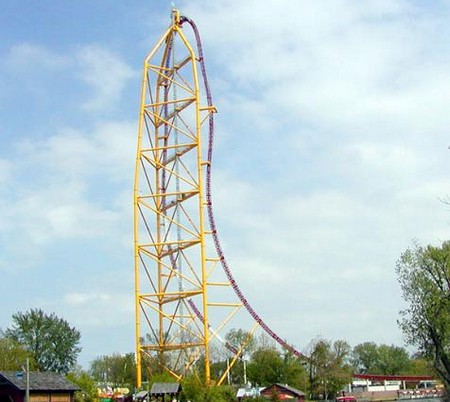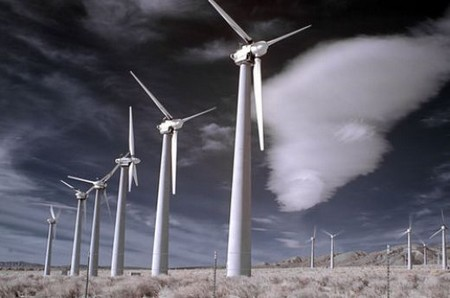## Types of Energy

Published on: Jan 01 2011 by Shenron

It is believed that the Universe that surrounds us exists in two forms that is, Substance and Force. Substance can be described by two basic aspects that is, mass and energy. Mass is defined as the measurement of inertia and gravitation, while energy is defined as the scalar measure of the substance movement. The term energy is well known by the majority of the people, but the concrete definition of the term is not yet analyzed. The definition given above is true but little ambiguous as substance can be described by more forms of movement.

However, energy is defined as the measure of a physical system. It describes the quality of changes and processes which take place in the Universe. Energy is an aspect that helps us to measure the unity and connection between the movement forms of substance and their lively capacity of mutual transformation.

Energy is perhaps the most vital concept that a man has discovered. With having the correct and accurate comprehension of the term energy, one cannot analyze different energetic systems and energetic processes.

Potential Energy

There is a concept that an object can store energy in its position. We can illustrate this point with the help of the heavy ball of a demolition machine. When the ball is held at an elevated position, it store energy. Let us as have another example of a drawn bow. Drawn bow can also store energy in its position. For instance, if we examine a bow in its usual position, we can see that there is no energy stored in a bow. However, when the position of the bow is altered or pulled back, it will now able to store energy by virtue of its position. This stored energy of position is known as ‘potential energy’. The stored energy possessed by an object is called potential energy.Kinetic Energy

The energy of motion is known as kinetic energy. Any object that has motion either in vertical or horizontal direction possesses a kinetic energy. Kinetic energy is of different types such as rotational kinetic energy (energy due rotational motion); vibrational kinetic energy (energy due to vibrational motion); and translational kinetic energy (energy due motion from one location to another). Let us take an example of translational kinetic energy. This type of kinetic energy depends upon two factors, i.e. the mass of the object and the speed of the object. Therefore, the kinetic energy of the moving body is given by the following equation:  Ek = ½ mv2.

Chemical Energy

The energy stored by the bonds and atoms that make up molecules is known as chemical energy. The bonds rearrange themselves when allow to react with other chemicals. Sometimes, the process of rearrangement of bonds requires some extra energy which they get from the nearby surroundings. Such reactions are known as ‘endothermic’. However, in some cases the chemicals release the excessive energy into the environment and heat it up, such reactions are known as ‘exothermic’.

The most apt example of the exothermic reaction is the burning of something where a chemical reaction takes place between different chemicals. Let us take an example of burning of wood, where a chemical reaction takes place between the molecules in the wood and the oxygen in the air. This process converts a lot of chemical energy into heat energy and light energy.

Electrical Energy

The presence and flow of an electric charge is known as electrical energy. The electrical energy can be seen in several different fields such as static electricity, lightning, or electromagnetic fields. Humans have discovered so many ways to store the electrical charge for later use. We can define the concept of electrical energy by using several different technologies like charge, current and potential.Nuclear Energy

Nuclear energy is defined as the large amount of energy which is produced during the process of fission or when the atoms split. This sort of energy produces an enormous amount of heat for when the atom fragments hit other atoms, they split and produce more heat and the process goes on till the last atom left. Nuclear power plants use this process to produce electricity.